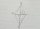# Trapezoid + expression of a variable from the formula - math problems

Trapezoid is a convex quadrilateral with one pair of parallel sides but referred to as a trapezium outside North America. The parallel sides are called the bases of the trapezoid, and the other two sides are called the legs or the lateral sides.

#### Number of examples found: 42

• TrapeziumThe area of trapezium is 35 cm2. Find its altitude if the bases are 6cm and 8 cm.
• GardenTrapezoid garden has parallel sides 19 m and 24 m. Its area is 193.5 square meters. What is the width of the garden?
• Isosceles trapeziumCalculate the area of an isosceles trapezium ABCD if a = 10cm, b = 5cm, c = 4cm.
• IsoscelesIsosceles trapezium ABCD ABC = 12 angle ABC = 40 ° b=6. Calculate the circumference and area.
• Trapezoid 15Area of trapezoid is 266. What value is x if bases b1 is 2x-3, b2 is 2x+1 and height h is x+4
• KLMN trapezoidThe KLMN trapezoid has bases KL 40cm and MN 16cm. On the KL base is point P. The segment NP divides the trapezoid into units with the same area. What is the distance of point P from point K?
• Trapezoidtrapezoid ABCD a = 35 m, b=28 m c = 11 m and d = 14 m. How to calculate its area?
• DitchDitch profile is an isosceles trapezoid with bases of length 80m and 60m. The slope of the side wall of the ditch is 80°. Calculate the ditch depth.
• Trapezium basesFind the trapezium height if a = 8 cm and c = 4 cm if its content 21 square centimeters.
• Trapezoid ABCD v2Trapezoid ABCD has length of bases in ratio 3:10. The area of riangle ACD is 825 dm2. What is the area of trapezoid ABCD?
• Trapezoid MO-5-Z8Trapezoid KLMN has bases 12 and 4 cm long. The area of triangle KMN is 9 cm2. What is the area of the trapezoid KLMN?
• Rectangular trapeziumCalculate the perimeter of a rectangular trapezium when its content area is 576 cm2 and sice a (base) is 30 cm, height 24 cm.
• Trapezoid thirdsThe ABCD trapezoid with the parallel sides of the AB and the CD and the E point of the AB side if the segment DE divides the trapezoid into two parts with the same area. Find the length of the AE line segment.
• The tractorThe tractor sows an average of 1.5 ha per hour. In how many hours does it sows a rectangular trapezoid field with the bases of 635m and 554m and a longer arm 207m?
• Rectangular trapezoidThe rectangular trapezoid ABCD is: /AB/ = /BC/ = /AC/. The length of the median is 6 cm. Calculate the circumference and area of a trapezoid.
• Isosceles trapezoidWhat is the height of an isosceles trapezoid, the base of which has a length of 11 cm and 8 cm and whose legs measure 2.5 cm?
• TrapezoidHow long are the trapezoid bases with area 24 cm2 and height 3 cm. One base is 3 times longer than the shorter.
• A kiteABCD is a kite. Angle OBC = 20° and angle OCD = 35°. O is the intersection of diagonals. Find angle ABC, angle ADC and angle BAD.
• TrenchThe trench is a four-sided prism. The cross section has a trapezoidal shape with basements of 4m and 6m, the length of the trench is 30m. What is the depth of the trench if we dig 60,000 l of soil.
• Land - isosceles trapezoidCalculate the content and perimeter of the building plot in the form of an isosceles trapezoid with bases 120m, 95m and height 50m.

Do you have an interesting mathematical word problem that you can't solve it? Submit a math problem, and we can try to solve it.

We will send a solution to your e-mail address. Solved examples are also published here. Please enter the e-mail correctly and check whether you don't have a full mailbox.

Please do not submit problems from current active competitions such as Mathematical Olympiad, correspondence seminars etc...